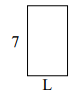# Finding the side length of a rectangle given its perimeter or area

In this lesson, we solve problems where we find one missing side length while one side length and area or perimeter of the rectangle are given.

Determine the value of 'L'.

Perimeter = 22 cm### Solution

Step 1:

Perimeter of a rectangle = 2(l + w); l = length = L; w = width = 6

Step 2:

Perimeter of given rectangle = 2(L + 6) = 22 cm

L + 6 = $\frac{22}{2}$ = 11;

So missing length L = 11 – 6 = 5 cm

Determine the value of 'L'.

Perimeter = 20 cm### Solution

Step 1:

Perimeter of a rectangle = 2(l + w); l = length = L; w = width = 7

Step 2:

Perimeter of given rectangle = 2(L + 7) = 20 cm

L + 7 = $\frac{20}{2}$ = 10;

So missing length L = 10 – 7 = 3 cm